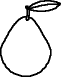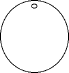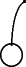EnchantedLearning.com is a user-supported site.
As a bonus, site members have access to a banner-ad-free version of the site, with print-friendly pages.

 You might also like: Divide Ten Pets Worksheet Printout Divide Fruit into Three Groups Worksheet Printout Divide Six by 2, 3, and 6 Worksheet Printout Divide Fish by Five Worksheet Printout Divide Eight Birds Worksheet Printout Today's featured page: Australia - Follow the Instructions

 Go to the AnswersMath Pages EnchantedLearning.com Divide Fruit into Four Groups Divide the fruit into groups to do division. Visual Division PagesDivision Pages

 Divide the pears into four equal groups.How many pears are there? How many groups of pears are there? How many pears are in each group?
4 ÷ 4 =

 Divide the oranges into four equal groups.How many oranges are there? How many groups of oranges are there? How many oranges are in each group?
8 ÷ 4 =

 Divide the cherries into four equal groups.How many cherries are there? How many groups of cherries are there? How many cherries are in each group?
12 ÷ 4 =

## Enchanted Learning Search

 Search the Enchanted Learning website for: